# iMA (mql4)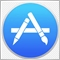35441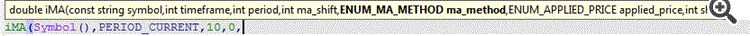In the shown pic above, Please let us know what is the difference between the (int ma_shift) in the center of function inputs & (int shift) in the last of function inputs ...

`iMA (Symbol(), PERIOD_D1, 14, 0, MODE_SMA, PRICE_CLOSE, 0);`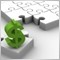10949

Mohammad Soubra:

In the shown pic above, Please let us know what is the difference between the (int ma_shift) in the center of function inputs & (int shift) in the last of function inputs ...

The MA shift is what it says - the bar shift that the MA uses when calculating. Have a look at the input options when you apply a simple MA to a chart. It is the shift function in here.

The last shift in iMA is the bar number you want to get the MA result from. So 0 is the current bar, 1 is the previous bar etc.35441

Stuart Browne:

The MA shift is what it says - the bar shift that the MA uses when calculating. Have a look at the input options when you apply a simple MA to a chart. It is the shift function in here.

The last shift in iMA is the bar number you want to get the MA result from. So 0 is the current bar, 1 is the previous bar etc.

Thanks Mr. Stuart

but...

plz look at the image below and let me know where are the twice of them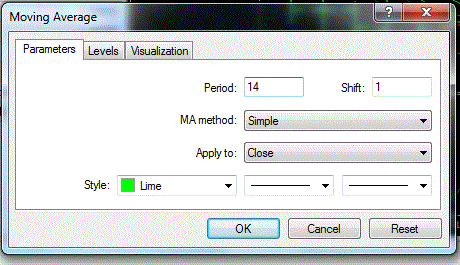I can see only one shift !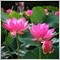53553

Below is 2 MA(14), red line used Shift =0, blue line used Shift= 10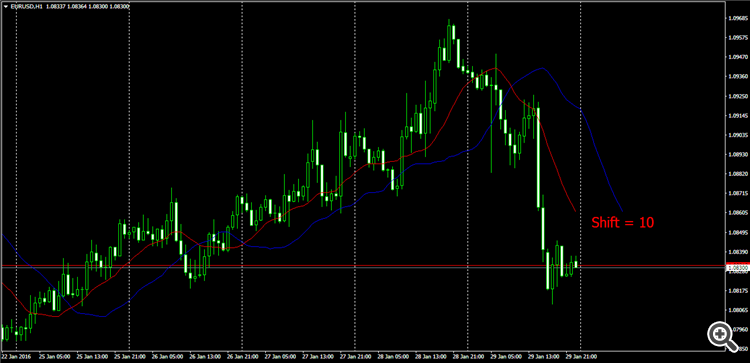35441

thanks Trinh,

the image you have been sent is like:

```iMA (Symbol(), PERIOD_D1, 14, 0, MODE_SMA, PRICE_CLOSE, 10);
iMA (Symbol(), PERIOD_D1, 14, 0, MODE_SMA, PRICE_CLOSE, 0);
```

//------------------------------

but if you use the code :

```iMA (Symbol(), PERIOD_D1, 14, 0, MODE_SMA, PRICE_CLOSE, 10);
iMA (Symbol(), PERIOD_D1, 14, 0, MODE_SMA, PRICE_CLOSE, 0);

iMA (Symbol(), PERIOD_D1, 14, 10, MODE_SMA, PRICE_CLOSE, 10);
iMA (Symbol(), PERIOD_D1, 14, 10, MODE_SMA, PRICE_CLOSE, 0);```

I cannot see that referred on the pic above while looking @ a chart35441

Stuart Browne:

The MA shift is what it says - the bar shift that the MA uses when calculating. Have a look at the input options when you apply a simple MA to a chart. It is the shift function in here.

The last shift in iMA is the bar number you want to get the MA result from. So 0 is the current bar, 1 is the previous bar etc.

please mr Stuart can you clarify more .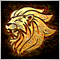Moderator
13500

The bar shift is not used in the input window because the visible portion will run over all bars on the chart creating the moving average line.

For a robot, however, if you want to know the value of a specific bar, that is where the last variable comes into play.

You have to specify a single buffer position.

In summary, the first shift shifts the entire calculation over the chart (All bars), while the last shift specifies the index value of the indicator buffer (A single bar).53553

Mohammad Soubra:

thanks Trinh,

the image you have been sent is like:

but if you use the code :

I cannot see that referred on the pic above while looking @ a chart

No,  MA Shift = 10 , see chart below

```   double ma1= iMA(NULL,0,14,0,MODE_SMA,PRICE_CLOSE,0);

double ma2= iMA(NULL,0,14,10,MODE_SMA,PRICE_CLOSE,0);

Comment("At Bar 0 ( or shift = 0 ) : MA1 = " + DoubleToStr(ma1,5) + " MA2 = " + DoubleToStr(ma2,5));
```35441

Marco vd Heijden:

The bar shift is not used in the input window because the visible portion will run over all bars on the chart creating the moving average line.

For a robot, however, if you want to know the value of a specific bar, that is where the last variable comes into play.

You have to specify a single buffer position.

In summary, the first shift shifts the entire calculation over the chart (All bars), while the last shift specifies the index value of the indicator buffer (A single bar).

Hello my friend :)

can you clarify imore n imagesModerator
13500

sure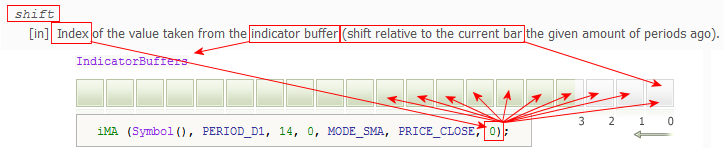35441

Marco vd Heijden:

sure

MY FRIEND !

This is not what I am exactly asking about

I am asking about the shift in the beginning

see the images below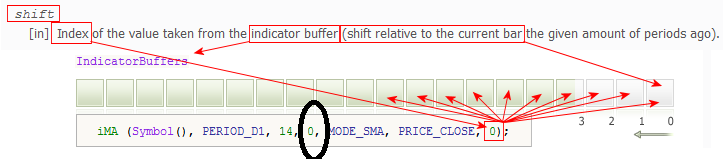or

or